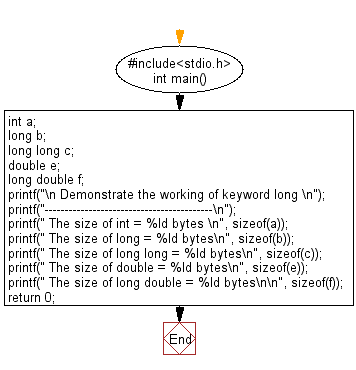﻿ C Program: Demonstrate the working of keyword long - w3resource

# C Exercises: Demonstrate the working of keyword long

## C Variable Type : Exercise-18 with Solution

Write a C program to demonstrate the working of the keyword long.

Sample Solution:

C Code:

``````#include<stdio.h>      // Include the standard input/output header file.

int main()           // Start of the main function.
{
int a;            // Declare an integer variable 'a'.
long b;           // Declare a long integer variable 'b'.
long long c;      // Declare a long long integer variable 'c'.
double e;         // Declare a double precision floating-point variable 'e'.
long double f;    // Declare a long double precision floating-point variable 'f'.

printf("\n Demonstrate the working of keyword long \n");   // Print a header message.
printf("------------------------------------------\n");  // Print a separator line.

printf(" The size of int = %ld bytes \n", sizeof(a));      // Print the size of 'a'.
printf(" The size of long = %ld bytes\n", sizeof(b));      // Print the size of 'b'.
printf(" The size of long long = %ld bytes\n", sizeof(c)); // Print the size of 'c'.

printf(" The size of double = %ld bytes\n", sizeof(e));    // Print the size of 'e'.
printf(" The size of long double = %ld bytes\n\n", sizeof(f)); // Print the size of 'f'.

return 0;   // Return 0 to indicate successful execution of the program.
}   // End of the main function.
```
```

Sample Output:

``` Demonstrate the working of keyword long
------------------------------------------
The size of int = 4 bytes
The size of long = 8 bytes
The size of long long = 8 bytes
The size of double = 8 bytes
The size of long double = 16 bytes
```

Flowchart:C Programming Code Editor:

What is the difficulty level of this exercise?

Test your Programming skills with w3resource's quiz.

﻿